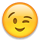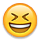## Forum Stats

• 3,825,238 Users
• 2,260,485 Discussions

Discussions

# String to Row: Delimiter as part of the value

My DB Version - 10.2.0.4.0

I have a string like this
```with t
as
(
from dual
)
select * from t```
I am looking for a SQL solution that will convert this string into row like this
```'My column'
10
10000```
Normal way to convert delimited string to row would be like this
```with t
as
(
from dual
)
select regexp_substr(str,'[^,]+',1,level) val
from t
connect by level <= length(str)-length(replace(str,','))+1```
But this would result in
```'My column'
4
'0')
10
10000 ```
But this is incorrect. So any idea how to solve it?

• Hi, Karthick,

Sorry I didn't reply earlier. I glanced at the thread list, saw that the last post was by "Karthick Arp", and thought "This thread is as good as answered already."

So you don't want to count delimiters that are inside parentheses.
One thing you can do is to split the string at all delimiters, then re-combine parts that are inside parentheses. We can tell if the end of the string is within parentheses by counting the number left parentheses that have occured up to that point, and subtracting the number of right parentheses.

In Oracle 11.1:
```with t
as
(
from dual
)
,	got_parts	AS
(
select regexp_substr(str,'[^,]+',1,level) val
,      LEVEL				  AS part_num
from t
connect by level <= length(str)-length(replace(str,','))+1
)
,	got_paren_cnt	AS
(
SELECT	got_parts.*
,	SUM ( REGEXP_COUNT (val, '\(')
- REGEXP_COUNT (val, '\)')
) OVER ( ORDER BY 	   part_num
ROWS BETWEEN  UNBOUNDED PRECEDING
AND	   1	     PRECEDING
)	AS paren_cnt
FROM	got_parts
)
SELECT	part_num
,	LTRIM ( SYS_CONNECT_BY_PATH (val, ',')
, ','
)		AS recombined_val
FROM	got_paren_cnt
WHERE	CONNECT_BY_ISLEAF	= 1
CONNECT BY	paren_cnt		!= 0
AND	part_num		= PRIOR part_num + 1
ORDER BY	part_num
;```
This will not handle quoted parentheses. for example,
`instr (my_column, ')'),10,10000`
gets treated as one item.

You might be able to handle things like this properly without PL/SQL, but I think you need a PL/SQL function would be a lot easier to write and maintain.
«1

• Hello Karthick,
I am not too good with REG_EXP things (comparitively to what i know).
But will the dirty trick of replace work for you?

{cod}
with t
as
(
from dual
)
select replace(regexp_substr(str,'[^,]+',1,level),'~',',') val
from t
connect by level <= length(str)-length(replace(str,','))+1
```Cheers!!!
Bhushan                                                                                                                                                                                                                                                                                                                                                                                                                                                                                                                                                                                                                                                                                                                                                                                                                                                        ```
• I don't have the luxury of modifying the source information```with t
as
(
from dual
)
, new_
as (
select substr (str, 1, instr (str, '(') + 1)||replace (regexp_substr (str, '\(.+\)'), ',', '~')
||substr (str, instr (str, ')', -1)) str
from t
)
select replace(regexp_substr(str,'[^,]+',1,level),'~',',') val
from new_
connect by level <= length(str)-length(replace(str,','))+1```
as in
```SQL> with t
2  as
3  (
5  from dual
6  )
7  , new_
8  as (
9  select substr (str, 1, instr (str, '(') + 1)||replace (regexp_substr (str, '\(.+\)'), ',', '~')

10         ||substr (str, instr (str, ')', -1)) str
11    from t
12  )
13  select replace(regexp_substr(str,'[^,]+',1,level),'~',',') val
14  from new_
15  connect by level <= length(str)-length(replace(str,','))+1
16  /

VAL
----------------------------------------------------------------------------------------------------
'My column'
10
10000```
• Hmm does not work. But thats a good idea given by Bhushan to replace the comma in the value with some other character.

But i guess this one fails if we have multiple values with comma.
```with t
as
(
from dual
)
, new_
as (
select substr (str, 1, instr (str, '(') + 1)||replace (regexp_substr (str, '\(.+\)'), ',', '~')
||substr (str, instr (str, ')', -1)) str
from t
)
select replace(regexp_substr(str,'[^,]+',1,level),'~',',') val
from new_
connect by level <= length(str)-length(replace(str,','))+1```
I think i need to match Brackets and do the replace in the source. Working on it• {noformat}*nod*{noformat}, once you start introducing delimiters that are also non delimiters then you get into the realms of recursive parsing of the string so that you only break on delimiters that are not nested inside brackets. It can get messy and often PL/SQL code is the simplest way to do it, but not the most efficient.
• I totally understand. But we should also accept the fact that at times we get such crazy stuff to work with.

Just hoping that some one comes up with a super cool sql to do this• Karthick_Arp wrote:
I totally understand. But we should also accept the fact that at times we get such crazy stuff to work with.

Just hoping that some one comes up with a super cool sql to do thisSomething like this perhaps?
```SQL> ed
Wrote file afiedt.buf

1  with t as (select q'['My column',LPAD(TRIM(my_column),4,'0'),10,10000]' str from dual)
2      ,x as (select regexp_substr(str, '[^(]+', 1, rownum) str, rownum as lvl
3             from t
4             connect by rownum <= length(regexp_replace(str, '[^(]'))+1
5            )
6      ,y as (select x.str as orig_str, lvl
7                   ,replace(regexp_replace(x.str, '[^\)]+\$'),',','~')||regexp_substr(x.str, '[^\)]+\$') as str2
8             from x
9            )
10      ,z as (select ltrim(sys_connect_by_path(str2, '('),'(') as str
11             from y
12             where connect_by_isleaf = 1
13             connect by lvl = prior lvl + 1
15            )
16  --
17  select rownum rn, replace(regexp_substr(str, '[^,]+', 1, rownum),'~',',') str
18  from z
19* connect by rownum <= length(regexp_replace(str, '[^,]'))+1
SQL> /

RN STR
---------- ----------------------------------------
1 'My column'
3 10
4 10000

SQL> ed
Wrote file afiedt.buf

1  with t as (select q'['My column',LPAD(TRIM(SUBSTR(my_column,4)),4,'0'),10,SUBSTR('FRED',4),10000]' str from dual)
2      ,x as (select regexp_substr(str, '[^(]+', 1, rownum) str, rownum as lvl
3             from t
4             connect by rownum <= length(regexp_replace(str, '[^(]'))+1
5            )
6      ,y as (select x.str as orig_str, lvl
7                   ,replace(regexp_replace(x.str, '[^\)]+\$'),',','~')||regexp_substr(x.str, '[^\)]+\$') as str2
8             from x
9            )
10      ,z as (select ltrim(sys_connect_by_path(str2, '('),'(') as str
11             from y
12             where connect_by_isleaf = 1
13             connect by lvl = prior lvl + 1
15            )
16  --
17  select rownum rn, replace(regexp_substr(str, '[^,]+', 1, rownum),'~',',') str
18  from z
19* connect by rownum <= length(regexp_replace(str, '[^,]'))+1
SQL> /

RN STR
---------- ----------------------------------------
1 'My column'
3 10
4 SUBSTR('FRED',4)
5 10000

SQL>```
Seems to work, but don't hold me to that.• Hi, Karthick,

Sorry I didn't reply earlier. I glanced at the thread list, saw that the last post was by "Karthick Arp", and thought "This thread is as good as answered already."

So you don't want to count delimiters that are inside parentheses.
One thing you can do is to split the string at all delimiters, then re-combine parts that are inside parentheses. We can tell if the end of the string is within parentheses by counting the number left parentheses that have occured up to that point, and subtracting the number of right parentheses.

In Oracle 11.1:
```with t
as
(
from dual
)
,	got_parts	AS
(
select regexp_substr(str,'[^,]+',1,level) val
,      LEVEL				  AS part_num
from t
connect by level <= length(str)-length(replace(str,','))+1
)
,	got_paren_cnt	AS
(
SELECT	got_parts.*
,	SUM ( REGEXP_COUNT (val, '\(')
- REGEXP_COUNT (val, '\)')
) OVER ( ORDER BY 	   part_num
ROWS BETWEEN  UNBOUNDED PRECEDING
AND	   1	     PRECEDING
)	AS paren_cnt
FROM	got_parts
)
SELECT	part_num
,	LTRIM ( SYS_CONNECT_BY_PATH (val, ',')
, ','
)		AS recombined_val
FROM	got_paren_cnt
WHERE	CONNECT_BY_ISLEAF	= 1
CONNECT BY	paren_cnt		!= 0
AND	part_num		= PRIOR part_num + 1
ORDER BY	part_num
;```
This will not handle quoted parentheses. for example,
`instr (my_column, ')'),10,10000`
gets treated as one item.

You might be able to handle things like this properly without PL/SQL, but I think you need a PL/SQL function would be a lot easier to write and maintain.
• REGEXP_COUNT is 11g. Karthik's on 10g.• Hi,
REGEXP_COUNT is 11g. Karthik's on 10g.Sorry, I missed that.
The only Oracle 11 feature I used was REGEXP_COUNT.
The standard work-around for REGEXP_COUNT (remove the character to be counted, then take the LENGTH) works nicely here.

This works in Oracle 10.1:
```with t
as
(
-- select q'[instr (my_column, ')'),10,10000]' str
from dual
)
,	got_parts	AS
(
select regexp_substr(str,'[^,]+',1,level) val
,      LEVEL				  AS part_num
from t
connect by level <= length(str)-length(replace(str,','))+1
)
,	got_paren_cnt	AS
(
SELECT	got_parts.*
,	SUM ( LENGTH (REPLACE (val, ')'))
- LENGTH (REPLACE (val, '('))
) OVER ( ORDER BY 	   part_num
ROWS BETWEEN  UNBOUNDED PRECEDING
AND	   1	     PRECEDING
)	AS paren_cnt
FROM	got_parts
)
SELECT	part_num
,	LTRIM ( SYS_CONNECT_BY_PATH (val, ',')
, ','
)		AS recombined_val
FROM	got_paren_cnt
WHERE	CONNECT_BY_ISLEAF	= 1